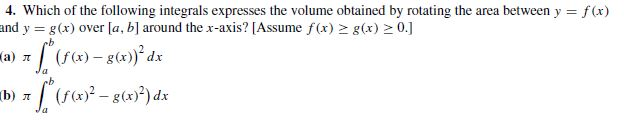# 4. Which of the following integrals expresses the volume obtained by rotating the area between y = f(x) and y = g(x) over [a, b] around the x-axis? [Assume f(x) > g(x) > 0.] L(r)-se)° dz |(F - 8(x)²) dx а) л b) л Ja

Questionhelp_outlineImage Transcriptionclose4. Which of the following integrals expresses the volume obtained by rotating the area between y = f(x) and y = g(x) over [a, b] around the x-axis? [Assume f(x) > g(x) > 0.] L(r)-se)° dz |(F - 8(x)²) dx а) л b) л Ja fullscreen